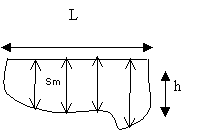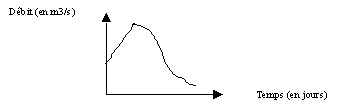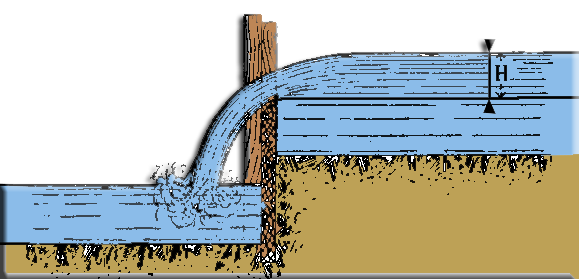measurement of river flows
We gather here some methods for measuring flow rates rivers.
• (http://www.risques.meteo.edu) :
River flow. - The measurement of the flow of a river is a delicate operation that requires some practice to achieve the proper accuracy.
Three methods may be used 1 the floater, 2 the valve, 3 the weir
Materials and preparation
• Bottle or piece of wood
• colored tracer
• water
• bucket
• chronometer
• Meter / tape measure (according to the study section)
• Calculator
• graph paper
• boots
1) Measuring the average flow velocity

according to the formula V= D/t with :
V : average speed m/s
D : distance between 2 points in meters
t : time in secondes

Choose a section of gauging where the water streams are parallel. For this remove all obstacles (stones, branches.) Included in the study area that locally modify the flow of rivers.

• Technique weighted float
Take a bottle filled with water or a piece of wood as a float
Define a starting point (1) and end point (2)
Measure the distance between these two points, then the note (D in meters)
Put the floater in the water, as centrally as possible, and measure the time it takes to reach the point 2
Calculating the average velocity.
• Tracer technique
Prepare a (non-polluting for the environment and preferably biodegradable) colored solution by mixing a dye in a bucket and water. The mixture should be colorful enough to be visible during its introduction into the watercourse (dissolution phenomenon)
Define a starting point (1) where the solution and make a point of arrival (2) when measuring time. The goal is to track the progress of the colored solution in the current. Measure the distance between these two points, and the note (D in meters) Pour the solution in 1, in the center of the stream
Timing the arrival time of the dye front in point 2 and the note (t in seconds)
Calculating the average velocity by using the formula
2) Measurement of the wetted surface
To know the morphology in the river at the study area (section gauging) in order to calculate the wet surface, it is necessary to take readings of the water level of the section gauging.
Measure the distance between the shores and to note (L in meters)
Take readings water depth using a graduated stick that is placed at the bottom of rivers. Make several measures the width of the river at regular intervals perpendicular to the shoreline. Note values ​​(h cm)
On a sheet of graph paper postpone the values ​​of h and model profile section
Calculate the wetted surface (Sm) with the grid and convert m²
Note Sm3) Calculation of the average flow of the river
• Apply the formula : Q = V x Sm with :
Q : flow rate m3/s
V :mean velocity in m/s
Sm : wetted surface of the study section inm²
at different times of the year (winter, spring.) and construct a hydrograph (flow vs. time)..Make comparisons and assumptions on flow variation (rainfall)
• site http://moulinafer.free.fr/ de Mr Robert Girard :

2°) Pressurized valve
- The way to determine the flow of rivers is convenient, because it is rare that manufacturing plants with a hydraulic force do not have a valve where I 'we can do this experiment. We lifts the valve, and the rule to the desired height, so that leaves this hole, just the amount of water to be measured (Figure 21). We is careful to let the water run for a while, to ensure that there is no decrease or increase in the upstream level, which would be evidence that the amount of water charged by opening the valve does not match the normal flow of the river.
The formula used to calculate the flow holes in a thin wall pressure is as follows:
• D = m . L . E . sqrt(2g . H)
Where : (D) is the flow rate in liters per second,
(L) discharge orifice width,
(E) height of the outlet opening,
(H) water pressure or load to the center of the orifice,
(g) 9.81,
(m)contraction coefficient ranging from 0,60 to 0,65 the form and the position of the orifice discharge.3°) Weir thin wall
- The weir is first and foremost a practical means of assessing the flow of a river ;
it is more used and accurate than the valve.The reservoir is formed by a thin vertical wall erected to the upper portion and tapered or beveled garnished sheet metal:
• The flow is given by the following formula :
D = m . L .H . sqrt(2g . H)
Where :
(D) is the flow rate in liters per second,
(L) tank width,
(H) load on the threshold, measured 1 m. 50 upstream,
sqrt(2g . H)  : theoretical flow speed,
(m) contraction coefficient ranging from 0,38 to 0,42 the form